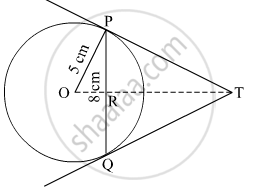# In Figure 2, PQ is a chord of length 8 cm of a circle of radius 5 cm. The tangents at P and Q intersect at a point T. Find the length TP. - Mathematics

Sum

In Figure 2, PQ is a chord of length 8 cm of a circle of radius 5 cm. The tangents at P and Q intersect at a point T. Find the length TP.#### Solution

PT = TQ (Tangents drawn from a common point to the circle are equal)
So, ΔTPQ is isosceles with PT = TQ and TO is thus the angle bisector of ∠PTQ.
So, OT  PQ and thus, OT bisects PQ. (Perpendicular drawn from the center of the circle to the chord bisects the chord)
Thus, PR = RQ = 4 cm
Also, in ΔOPR,

OR =sqrt("OP"^2-"PR"^2)

=sqrt(5^2 - 4^2)

= 3 cm

In ΔTPR,

TR2 + PR2 = TP2

⇒ TR2 + 42 = TP2

⇒ TR2 + 16 = TP2

Let TP = x and TR = y

⇒ Y2 + 16 = X2 .....(1)

In Δ OPT,

TP2 + OP2 = OT2

⇒ TP2 + 52 =  (TR + 3)2

⇒ X2 + 25 = (Y + 3)2.................(2)

Using (1) and (2) we get

y =16/3

putting this value of y in (1) we get

(16/3)^2 + 16 = "x"^2

⇒ 256/9 + 16 = "x"^2

⇒ (256+144)/9 ="x"^2

⇒ 400/9 = "x"^2

⇒ "x" = 20/3

Thus, the length of TP =20/3

Concept: Construction of Tangents to a Circle
Is there an error in this question or solution?